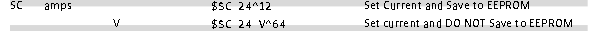Start a new topic

## RAPI checksums/carrige ID?

I am trying to figure out how to execute "\$SC xx V" RAPI commands, using the correct checksum, so I can properly interpret the response using a script. I am a bit baffled how to calculate this though, and was hoping for some explanation:

\$= start of RAPI command

cc = 2-letter command

pp = parameters

xk = 2-hex-digit checksum - 8-bit XOR of all characters before '^'

ss = optional 2-hex-digit sequence id - response will echo the

sequence id - ss CANNOT be 00, which is reserved as an

invalid value

\r = carriage return = 13d = 0x0D

Checksum + Sequence ID

\$cc pp :ss^xk\r

I understand how to structure the body of the command, up until the ^xk\r

Can someone explain, for example, how I would know that the proper flag to attach to this command, from the OpenEVSE documentation, is ^64?How would I go about figuring out the flag to attach to \$SC 12 V or any other current rating?